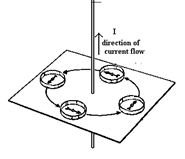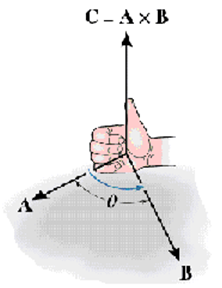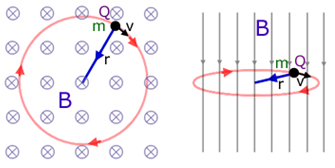# Moving Charges and Magnetism - Online Test

Q1. A wire is perpendicular to the plane of the paper. A ring of compass needles surrounds the wire in the plane of the paper with center of ring being the center of the wire .Initially there is no current in the wire. What happens after a steady dc current is established in the wire?
Explaination / Solution:

The current carrying wire has a magnetic field around it and the lines of force are in the form of concentric circles with their centers on the wire.Q2. Which of the following is most general charachteristic of Magnetic field
Explaination / Solution:

A moving charge experiences a force in a magnetic field called magnetic Lorentz force given by.This force acts only if the particle (i) has charge q(ii) has a non zero velocity v and (iii) does not move parallel or anti parallel to the magnetic field B. A current carrying conductor placed in a magnetic field experiences a force given by
Q3. which of the following best describes the difference between electric and magnetic fields
Explaination / Solution:

The electrostatic force experienced by a positive charge q placed in an electric field is . The force acts in the direction of the electric field.The magnetic force acting on a charge of magnitude q moving with a velocity 'v' is .This acts in a direction perpendicular to the plane containing the vectors.$\stackrel{}{v}$ and
Q4. direction of magnetic force on a positive charge moving in a magnetic field is given by
Explaination / Solution:

The force experienced by a charged particle in a magnetic field is given by .The right hand rule for vector product can be used to determine the direction of $\stackrel{}{F}$.Q5. Lorentz Force generally refers to
Explaination / Solution:

A moving charge experiences a force in a magnetic field called magnetic Lorentz force given by
Q6. correct unit of magnetic field is
Explaination / Solution:

The SI unit of magnetic field is Tesla (T)

Q7. correct expression for force on a current carrying conductor of length dl in a magnetic field is
Explaination / Solution:

force experienced by a conductor of length l carrying current I placed in magnetic field B is given by .Here $\stackrel{\to }{l}$=

Q8. The magnitude of the magnetic field due to a circular coil of radius R carrying a current I at an axial distance x from the centre is
Explaination / Solution:

magnetic field at a point located at a distance x from the center of the coil of radius R carrying current along its axis is
Q9. Consider the motion of a charged particle in a uniform magnetic field directed into the paper.If velocity v of the particle is in the plane of the paper the charged particle will
Explaination / Solution:

The charged particle experiences magnetic Lorentz force given by.This force acts in the plane of the paper perpendicular to the velocity vector at all points on the plane. Magnetic Lorentz force acts like a centripetal force, causing the particle to move in a circle.Q10. cyclotron is used to
Explaination / Solution:

Cyclotron is a particle accelerator, which uses electric field to accelerate the charged particle and magnetic field to make it move in a circular path. During acceleration in the electric field, the particle acquires high energies of the order of a few million electron volts.